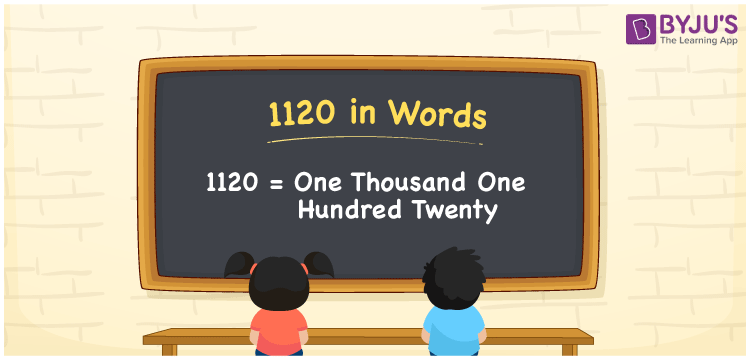# 1120 in Words

We can write the number 1120 in words as One Thousand One Hundred Twenty. For example, Sunita bought a dress worth Rs. 1120, then you can say, “Sunita bought a dress worth Rupees One Thousand One Hundred Twenty”.The conversion of numbers in word form is easy by using a place value method. 1120 is a cardinal number since it shows a specific quantity. Learn how to write and spell the number name of 1120 in English here.

 1120 in Words One Thousand One Hundred Twenty One Thousand One Hundred Twenty in numerical form 1120

## 1120 in English Words

Generally, we write numbers in words with the help of the English alphabet. Thus, the number 1120 in English is written as One Thousand One Hundred Twenty.## How to Write 1120 in Words?

Conversion of numbers into words is very easy using a place value chart. Here, 1120 is a four-digit number. Hence, we should make a chart of 4 columns to represent the place value chart for the number 1120.

 Thousands Hundreds Tens Ones 1 1 2 0

Hence, we can write the expanded form as:

1 x Thousand + 1 x Hundred + 2 x Ten + 0 x One

= 1 x 1000 + 1 x 100 + 2 x 10 + 0 x 1

= 1000 + 100 + 20 + 0

= 1000 + 100 + 20

= 1120

= One Thousand One Hundred Twenty

Therefore, 1120 in words is written as One Thousand One Hundred Twenty

Interesting way of writing 1120 in words

1 = One

11 = Eleven

112 = One Hundred and Twelve

1120 = One Thousand One Hundred Twenty

Thus, the word form of the number 1120 is One Thousand One Hundred Twenty

1120 is a natural number that is the successor of 1119 and the predecessor of 1121

• 1120 in words – One Thousand One Hundred Twenty
• Is 1120 an odd number? – No
• Is 1120 an even number? – Yes
• Is 1120 a perfect square number? – No
• Is 1120 a perfect cube number? – No
• Is 1120 a prime number? – No
• Is 1120 a composite number? – Yes

## Frequently Asked Questions on 1120 in Words

Q1

### How do you write 1120 in words?

1120 in words is written as One Thousand One Hundred Twenty.
Q2

### Simplify 1000 + 120, and express in words.

Simplifying 1000 + 120, we get 1120. Thus, 1120 in words is One Thousand One Hundred Twenty.
Q3

### Is 1120 an odd number?

No, the number 1120 is not an odd number.